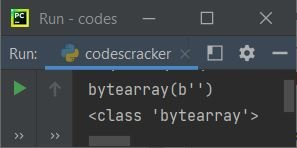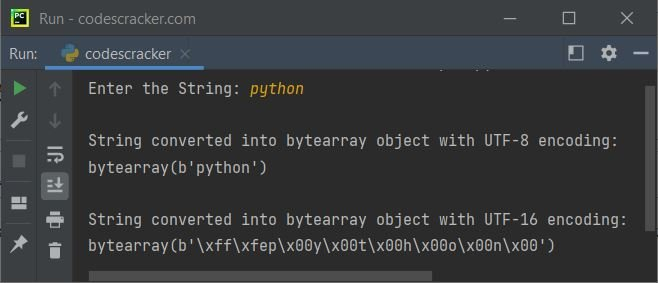# bytearray in Python

bytearray in Python is a binary sequence type. There are 3 binary sequence types available in Python:

1. bytes
2. bytearray
3. memoryview

The "bytearray" inbuilt type is used to manipulate binary data. Unlike bytes, bytearray objects are mutable, or changeable.

## Creating a bytearray in Python

A bytearray object can be created in four ways. All four ways of creating a bytearray object in Python are given below.

#### Using the bytearray() constructor

The bytearray() constructor is always required to create a bytearray object in Python. Here is an example:

```x = bytearray()
print(x)
print(type(x))```

The output of this Python program is shown in the picture below. It shows the value and type of the variable x:#### Creating a bytearray of a specified length

Here is another example of creating a bytearray object with a specified length:

```x = bytearray(5)
print(x)
print(type(x))```

The following is the output produced by this Python code:

```bytearray(b'\x00\x00\x00\x00\x00')
<class 'bytearray'>```

#### Creating a bytearray from an iterable

Here is the third example of creating a bytearray from an iterable:

```x = bytearray(range(5))
print(x)
print(type(x))```

The output should be:

```bytearray(b'\x00\x01\x02\x03\x04')
<class 'bytearray'>```

#### Copying Existing Binary Data via the Buffer Protocol

Here is the last example of creating a bytearray object by copying existing binary data via the buffer protocol:

```x = bytearray(b'codescracker dot com')
print(x)
print(type(x))```

The output should be:

```bytearray(b'codescracker dot com')
<class 'bytearray'>```

## Python bytearray example

This program is created to allow the user to enter a string. The entered string gets converted into a bytearray object using bytearray() and the utf-8 and  utf-16 encodings. You can also experiment with different encodings on your own.

```print("Enter the String: ", end="")
str = input()

print("\nString converted into bytearray object with UTF-8 encoding:")
ba = bytearray(str, "UTF-8")
print(ba)

print("\nString converted into bytearray object with UTF-16 encoding:")
ba = bytearray(str, "UTF-16")
print(ba)```

The snapshot given below shows the sample run with user input "python":## Python bytearray objects are mutable

Because bytearray objects are mutable, we can modify the bytes object anytime in the same program. Here is an example:

```str = "codescracker dot com"
x = bytearray(str, "utf-8")
print(x)

del x[12:]
print(x)

x[12:] = b".com"
print(x)```

In the above program, using the statement:

`del x[12:]`

All characters from index 12 (included) to the end will be deleted, and using the following statement:

`x[12:] = b".com"`

The new value, .com, will be appended to or replaced from the index 12th. Here is the sample output produced by the above Python program:

```bytearray(b'codescracker dot com')
bytearray(b'codescracker')
bytearray(b'codescracker.com')```

Note: To learn about slicing in detail, refer to the list slicing in Python post. There, you'll get all the details.

Check out the Python bytearray to string program to learn how to change a bytearray object into a string object.

Python Online Test

« Previous Tutorial Next Tutorial »